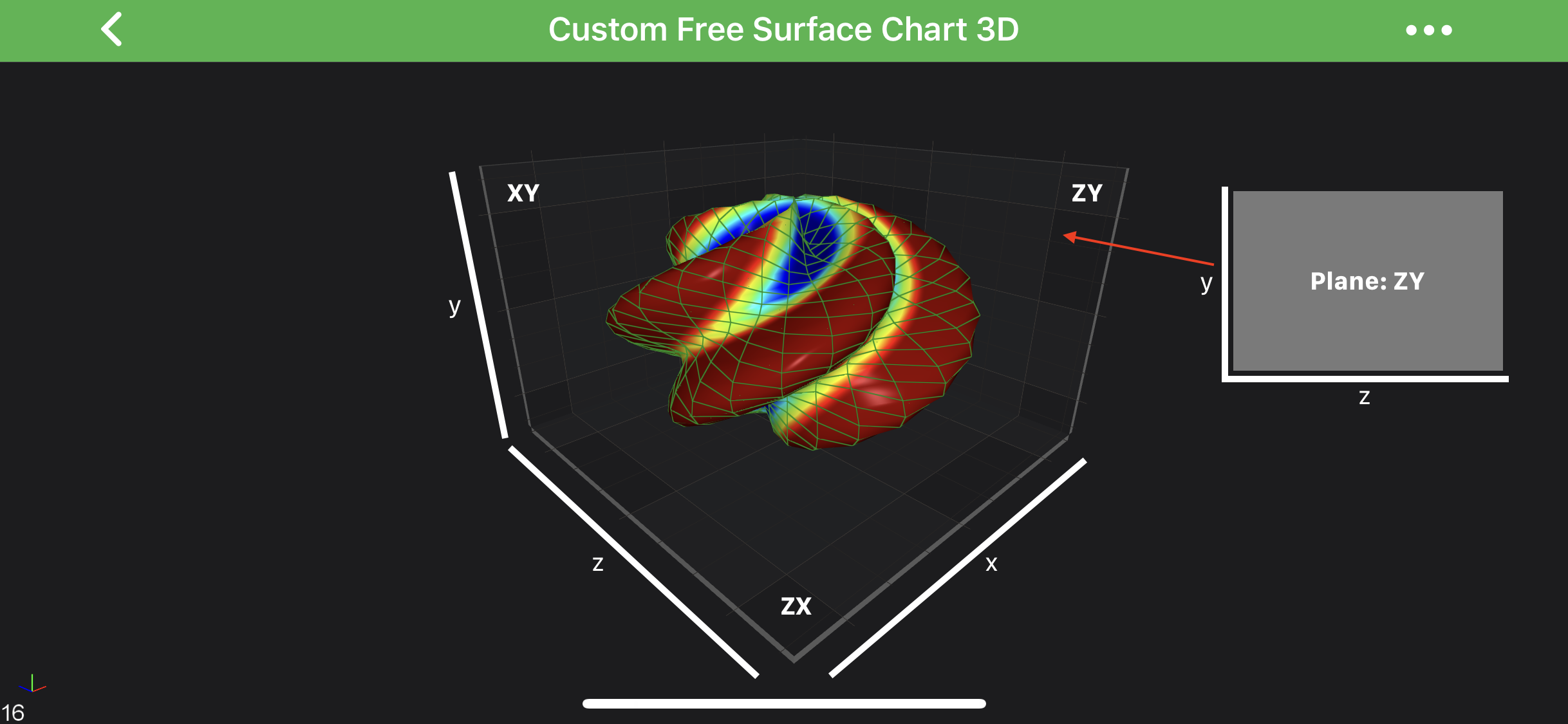iOS & macOS Charting Documentation - SciChart iOS & macOS Charts SDK v4.x

# Axis 3D Labels - Labels Configuration

In SciChart 3D you can configure showing the Axis Labels differently on the Axis Cube Planes. Possible options are defined by the `SCIAxisPlaneDrawLabelsMode` enumeration.

But first, let’s explain Axis Cube planes properly.

#### Axis Cube PlanesAs you might guess, there are three possible planes in axis cube, and those are defined as follows:

AxisCube Plane Description
XY Plane perpendicular to the Z-Axis (Left by default).
ZY Plane perpendicular to the X-Axis (Right by default).
ZX Plane perpendicular to the Y-Axis (up).

Let’s see the ZY Plane on the image below:#### Axis Cube Labels Configuration

As mentioned above, drawing labels are controlled by the `SCIAxisPlaneDrawLabelsMode` enumeration, which has the following options:

`SCIAxisPlaneDrawLabelsMode` Description
Both axis labels are drawn on both sides of the axis cube. This is the default mode.
Hidden hides the axis labels for this plane, for example, when applied to the XyAxisPlane, the labels drawn by this plane will be hidden.
LocalX draws the labels on one side - the local X - of the plane.
LocalY draws the labels on the other side - the local Y - of the plane.

The above modes can be applied to one of the AxisCube’s planes which are accessible through the following properties on the `SCIChartSurface3D`:

• `SCIChartSurface3D.xyAxisPlaneDrawLabelsMode`
• `SCIChartSurface3D.zyAxisPlaneDrawLabelsMode`
• `SCIChartSurface3D.zxAxisPlaneDrawLabelsMode`

See how it works in the code snippet below:

self.surface.xyAxisPlaneDrawLabelsMode = SCIAxisPlaneDrawLabelsMode_Both; self.surface.zyAxisPlaneDrawLabelsMode = SCIAxisPlaneDrawLabelsMode_LocalX; self.surface.zxAxisPlaneDrawLabelsMode = SCIAxisPlaneDrawLabelsMode_Hidden;
surface.xyAxisPlaneDrawLabelsMode = .both surface.zyAxisPlaneDrawLabelsMode = .localX surface.zxAxisPlaneDrawLabelsMode = .hidden
Surface.XyAxisPlaneDrawLabelsMode = SCIAxisPlaneDrawLabelsMode.Both; Surface.ZyAxisPlaneDrawLabelsMode = SCIAxisPlaneDrawLabelsMode.LocalX; Surface.ZxAxisPlaneDrawLabelsMode = SCIAxisPlaneDrawLabelsMode.Hidden;

Which results with the following: